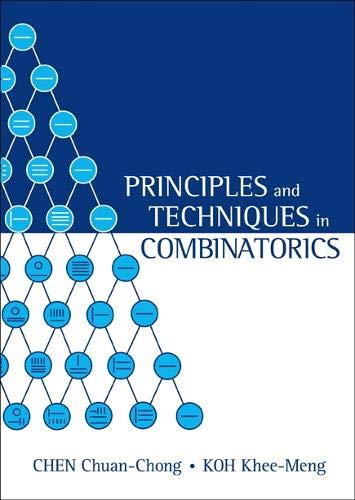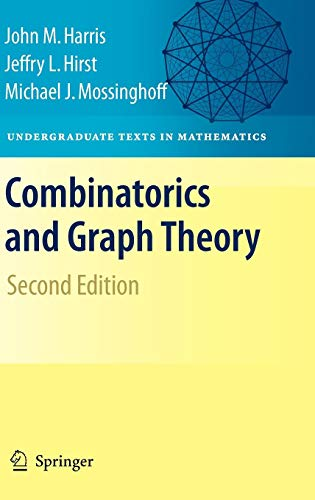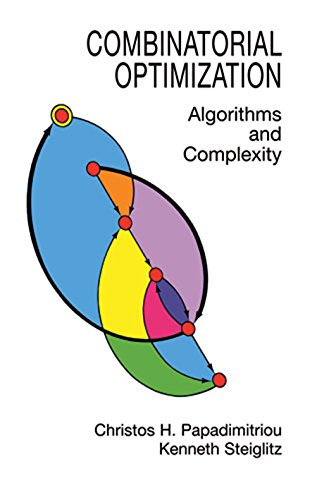# Best Combinatorics Books for Problem Solving

Combinatorics is the branch of mathematics dealing with combinations of objects belonging to a finite set in accordance with certain constraints, such as those of graph theory. The entire modern world relies on combinatorial algorithms. If you want to make a program faster, you need combinatorics. If you want to understand modern programming, you need combinatorics. Without combinatorics, some programs that now take a split second would require weeks.

Another vital application of combinatorics is in representation theory, symmetric functions, and the study of varieties with lots of symmetries (Grassmannians, flag varieties, toric varieties, symmetric varieties, spherical varieties. Here you will get some of the best Combinatorics Books

## 1. Principles and Techniques in CombinatoricsAuthor: KHEE-MENG KOH,CHUAN CHONG CHEN
Published at: 01/07/1992
ISBN: 9810211392

A useful book on Combinatorics. Concepts are well discussed and also has a nice example set. This Combinatorics book can be used by students preparing for math competitions. Very well written and easy to follow.

This book features:

• Permutations and Combinations.
• Binomial Coefficients and Multinomial Coefficients.
• The Pigeonhole Principle and Ramsey Numbers.
• The Principle of Inclusion and Exclusion.
• Generating Functions.
• Recurrence Relations.

## 2. Walk Through Combinatorics, A: An Introduction To Enumeration And Graph Theory (3Rd Edition)

This Walk Through Combinatorics book goes step by step on the elementary subjects of Combinatorics, contains many examples and has solutions of every example. This book is nicely organized and easy to understand.

This book includes:

• The Pigeon-Hole Principle.
• The Method of Mathematical Induction.
• Permutations.
• The Binomial Theorem and Related Identities.
• Partitions and Cycles in Permutations.
• The Sieve Formula and its applications.
• Ordinary and Exponential Generating Functions.
• The Origins of Graph Theory.
• Trees, Coloring, and Matching.
• Euler's Theorem for Planar Graphs.
• Ramsey Theory.
• Subsequent Conditions on Permutations.
• The Probabilistic Method.
• Partial Orders and Lattices.
• Block Designs and Error-Correcting Codes.
• Counting Unlabelled Structures.
• Combinatorial Algorithms.
• Computational Complexity.

## 3. Combinatorics and Graph Theory (Undergraduate Texts in Mathematics)Author: John Harris,Jeffry L. Hirst,Michael Mossinghoff
Published at: 19/09/2008
ISBN: 0387797106

This book offers:

• Graph Theory and Trees.
• Trails, Circuits, Paths, and Cycles.
• Planarity.
• Colorings and Matchings.
• Ramsey Theory.
• Binomial Coefficients and Multinomial Coefficients.
• The Pigeonhole Principle.
• Generating Functions.
• Polya's Theory of Counting.
• Ordinals, Cardinals and Many Pigeons.
• Combinatorial Geometry.
• Infinite Combinatorics and Graphs.
• Finite Combinatorics with Infinite Consequences.
• References.

## 4. Combinatorial Optimization: Algorithms and Complexity (Dover Books on Computer Science)Published at: 26/04/2013
ISBN: B00C8UQZAO
This book is rife with clear examples. The writing is clear, and the figures are informative and plentiful. All chapters have problem sets and notes and references. This book has a mighty amount of information, and it is amazingly well-explained. That's why this is one of the best combinatorics books.

This book delivers:

• Optimization Problems.
• The Simplex Algorithm.
• Duality.
• Computational Considerations for the Simplex Algorithm.
• The Primal-Dual Algorithm.
• Ford-Fulkerson and Dijkstra.
• Primal-Dual Algorithm for Min-Cost Flow.
• Algorithms and Complexity.
• Efficient Algorithms for the Max-Flow Problems.
• Algorithms for Matching.
• Weighted Matching.
• Spanning Trees and Matroids.
• Integer Linear Programming.
• A Cutting Plane Algorithm for Integer Linear Programs.
• NP-Complete Problems.
• Approximations Algorithms.
• Branch-and-Bound and Dynamic Programming.
• Local Search.

## 5. Combinatorics: A Very Short Introduction (Very Short Introductions)

A beautifully written book and easy to understand for everyone. It familiarizes with the basics of each topic first, and then gradually raises the bar. The examples are well concise and chosen perfectly.

This book contains: